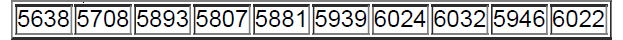×
Get Full Access to Elementary Statistics - 12 Edition - Chapter 2.4 - Problem 5re
Get Full Access to Elementary Statistics - 12 Edition - Chapter 2.4 - Problem 5re

×

# CO Emissions Listed below are the amounts (million metricISBN: 9780321836960 18

## Solution for problem 5RE Chapter 2.4

Elementary Statistics | 12th Edition

• Textbook Solutions
• 2901 Step-by-step solutions solved by professors and subject experts
• Get 24/7 help from StudySoup virtual teaching assistantsElementary Statistics | 12th Edition

4 5 1 363 Reviews
29
5
Problem 5RE

CO Emissions Listed below are the amounts (million metric tons) of carbon monoxide emissions in the United States for each year of a recent ten-year period. The data are listed in order. Construct the graph that is most appropriate for these data. What type of graph is best? What does the graph suggest?Step-by-Step Solution:
Step 1 of 3

Problem 5RE

Step1 of 3:

We have Listed below are the amounts (million metric tons) of carbon monoxide emissions in the United States for each year of a recent ten-year period. The data are listed in order.

 5638 5708 5893 5807 5881 5939 6024 6032 5946 6022

Step2 of 3:

We need to Construct the graph that is most appropriate for these data. What type of graph is best? What does the graph suggest?

Step3 of 3:

A frequency line chart is obtained by using Excel and steps are as followsEnter the given data in Excel sheetselect data(frequency column)go to insertchoose line graphthen press okA line graph, with the year number of the horizontal axis and the amount of carbon monoxide emissions on the vertical axis.

The graph suggest that the CO Emissions increase over the years because in a above line chart we can say that the value of CO emission increasing according as year.

Step 2 of 3

Step 3 of 3

##### ISBN: 9780321836960

Unlock Textbook Solution